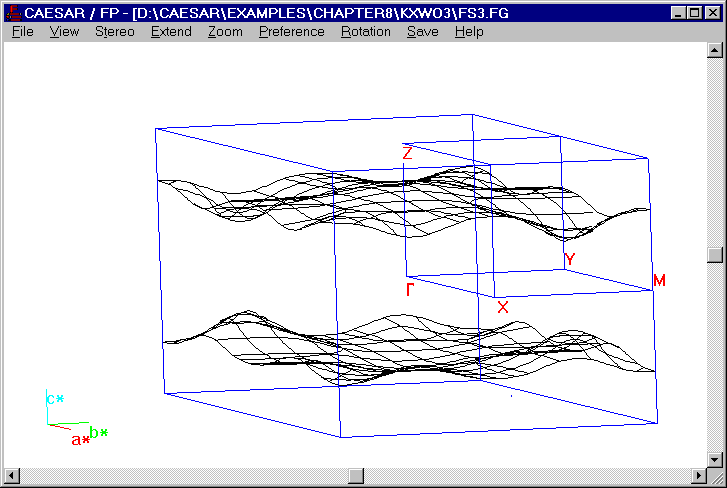# 3D Fermi Surface of WO3

The calculated Fermi surface nesting vector q = ~0.5c* is consistent with the observed superlattice reflection peaks of AxWO3 (A = K, Rb). However, CsxWO3 does not undergo a CDW transition although it has a 1D Fermi surface as do KxWO3 and RbxWO3. To understand this difference, one should note that a CDW formation involves a periodic lattice distortion, which strains the lattice and hence destabilizes it.11,12 Thus, a lattice cannot undergo a CDW transition unless the electronic energy gain dominates over the destabilization caused by the lattice strain. A CDW formation would be favorable for a system with soft lattice. As the lattice becomes stiffer, the CDW formation would become less favorable and hence occur at a lower temperature. The crystal structure of AxWO3 (A = K, Rb, Cs) suggests that the stiffness of the 3D WO3 lattice should increase as more alkali atom sites are occupied, and that for a given x value the stiffness of the lattice should increase in the order KxWO3 < RbxWO3 < CsxWO3, because the cation size increases in the order K+ < Rb+ < Cs+. The lack of a CDW transition in CsxWO3 implies that its lattice is too stiff to accommodate a CDW formation. The occurrence of a CDW in KxWO3 and RbxWO3 is consistent with the assertion that their lattices are less stiff than that of CsxWO3.Figure. Fermi surfaces of the [WO3]x- lattice

Go back to The Gallery